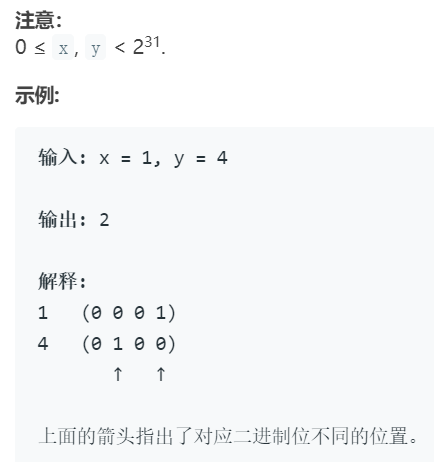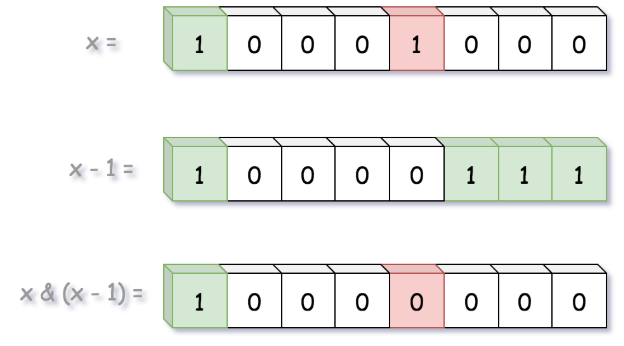# #461 汉明距离### 方法一：内置位计数功能

``````class Solution {
public int hammingDistance(int x, int y) {
return Integer.bitCount(x ^ y);
}
}
``````

### 方法二：移位

``````class Solution {
public int hammingDistance(int x, int y) {
int z = x ^ y;
int distance = 0;
while(z != 0){
if(z % 2 == 1){
distance +=1;
}
z = z >> 1;
}
return distance;
}
}``````

### 方法三：布赖恩·克尼根算法``````class Solution {
public int hammingDistance(int x, int y) {
int z = x ^ y;
int distance = 0;
while(z != 0){
distance += 1;
z = z & (z-1);
}
return distance;
}
}``````

THE END